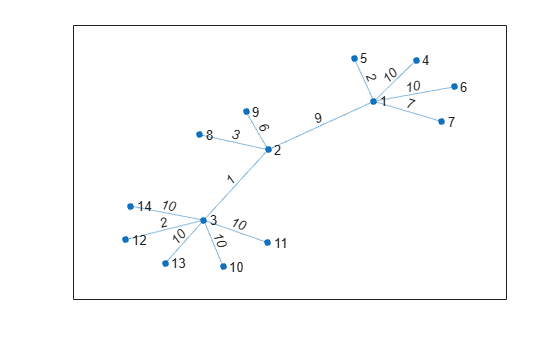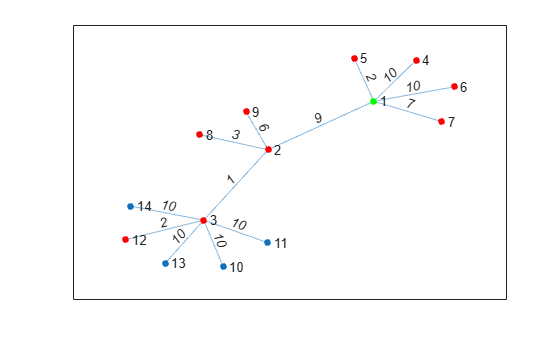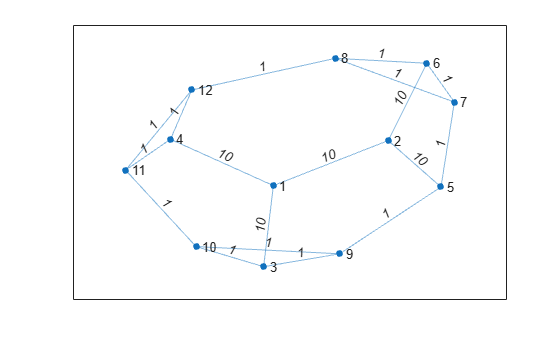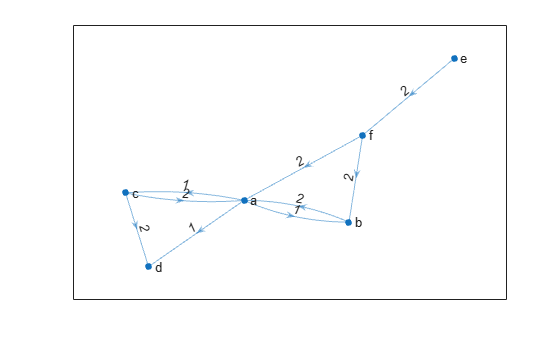# nearest

## 语法

``nodeIDs = nearest(G,s,d)``
``nodeIDs = nearest(G,s,d,Name,Value)``
``[nodeIDs,dist] = nearest(___)``

## 说明

``nodeIDs = nearest(G,s,d)` 返回图形 `G` 中与节点 `s` 的距离在 `d` 之内的所有节点。如果图进行了加权（即 `G.Edges` 包含变量 `Weight`），则这些权重用作沿图中各边的距离。否则，所有边距离都视为 `1`。`

``nodeIDs = nearest(G,s,d,Name,Value)` 使用一个或多个名称-值对组参数指定的其他选项。例如，如果 `G` 是加权图，则 `nearest(G,s,d,'Method','unweighted')` 将忽略图 `G` 中的边权重，而将所有边权重视为 `1`。`

``[nodeIDs,dist] = nearest(___)` 还返回与每个最近邻点之间的距离，因此 `dist(j)` 是从源节点 `s` 到节点 `nodeIDs(j)` 的距离。您可以使用上述语法中的任何输入参数组合。`

## 示例

```s = [1 1 1 1 1 2 2 2 3 3 3 3 3]; t = [2 4 5 6 7 3 8 9 10 11 12 13 14]; weights = randi([1 10],1,13); G = graph(s,t,weights); p = plot(G,'Layout','force','EdgeLabel',G.Edges.Weight);````nn = nearest(G,1,15)`
```nn = 9×1 5 7 2 3 4 6 8 12 9 ```

```highlight(p,1,'NodeColor','g') highlight(p,nn,'NodeColor','r')``````s = [1 1 1 2 2 6 6 7 7 3 3 9 9 4 4 11 11 8]; t = [2 3 4 5 6 7 8 5 8 9 10 5 10 11 12 10 12 12]; weights = [10 10 10 10 10 1 1 1 1 1 1 1 1 1 1 1 1 1]; G = graph(s,t,weights); plot(G,'EdgeLabel',G.Edges.Weight)````[nn,dist] = nearest(G,3,5)`
```nn = 9×1 9 10 5 11 4 7 12 6 8 ```
```dist = 9×1 1 1 2 2 3 3 3 4 4 ```

```s = {'a' 'a' 'a' 'b' 'c' 'c' 'e' 'f' 'f'}; t = {'b' 'c' 'd' 'a' 'a' 'd' 'f' 'a' 'b'}; weights = [1 1 1 2 2 2 2 2 2]; G = digraph(s,t,weights); plot(G,'EdgeLabel',G.Edges.Weight)````nn_out = nearest(G,'a',1)`
```nn_out = 3x1 cell {'b'} {'c'} {'d'} ```

`nn_in = nearest(G,'a',Inf,'Direction','incoming')`
```nn_in = 4x1 cell {'b'} {'c'} {'f'} {'e'} ```

## 输入参数

### 名称-值参数

`'outgoing'`（默认值）距离是使用源节点 `s`出向路径计算的。
`'incoming'`距离是使用源节点 `s`入向路径计算的。

`'auto'`（默认值）

`'auto'` 选项会自动选择算法：

• `'unweighted'` 用于没有边权重的 `graph``digraph` 输入。

• `'positive'` 用于具有边权重的所有 `graph` 输入，并要求权重为非负数。此选项还用于具有非负边权重的 `digraph` 输入。

• `'mixed'` 用于其边权重包含某些负值的 `digraph` 输入。图不能包含负循环。

`'unweighted'`

`'positive'`

Dijkstra 算法，要求所有边权重均为非负数。

`'mixed'`（仅适用于 `digraph`